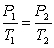Try out our new practice tests completely free!# Introductory Chemistry Study Set 7

Chemistry

## Quiz 6 : GasesStudy FlashcardsLooking for Introductory / Preparatory Chemistry Homework Help?

## Quiz 6 :GasesThe SI unit of pressure is atmosphereis defined as exactly 760 mmHg. (atm).
Free
True False

False

Tags
Choose question tagAvogadro's law states that equal volumes of different gases at the same temperature and pressure contain the same amount of gas.
Free
True False

True

Tags
Choose question tagGay-Lussac's law relates volume of a gas with absolute temperature.
Free
True False

False

Tags
Choose question tagWhen applying Charles's law, amount of gas and volume are considered to be constants.
True False
Tags
Choose question tagA sample of a gas has an initial volume of 15.0 L and an initial temperature of 235 K. The temperature of the gas increases to 313 K, if its volume increases to 20.0 L.
True False
Tags
Choose question tagP1T1 = P2T2, represents the Charles's law of gases.
True False
Tags
Choose question tagReal gases are ideal.
True False
Tags
Choose question tagOne torr is an equivalent unit of one millimeter of mercury, the amount of pressure exerted by a column of mercury exactly 1 mm high.
True False
Tags
Choose question tagAccording to Gay-Lussac's law,at constant V and n.
True False
Tags
Choose question tagA 6.00 mol sample of O2 has a pressure of 0.80 atm and a temperature of 68°C. Its volume is 41.8L.
True False
Tags
Choose question tagRobert Boyle invented the mercury barometer.
True False
Tags
Choose question tagThe density of O2 is 1.54 g/L at 30°C and 1.20 atm.
True False
Tags
Choose question tagIf a 2.45 L volume of gas contains 4.5 × 1021 gas particles, a 4.9 L volume of gas will contain 4.5 × 1022 gas particles.
True False
Tags
Choose question tagAn ideal gas is a gas that exactly follows the statements of the kinetic theory.
True False
Tags
Choose question tagPressure and volume of a gas are inversely related, if other parameters are kept the same.
True False
Tags
Choose question tagPascal (Pa) is equal to 1 N/m2.
True False
Tags
Choose question tagA sample of a gas has an initial volume of 16.8 L and an initial temperature of 50°C. The volume of the gas increases to 33.6 L if its temperature is increased to 100° C.
True False
Tags
Choose question tagThe equation, PV = nRT is called the ideal gas law, a gas law that relates all four independent physical properties of a gas under any conditions..
True False
Tags
Choose question tagAccording to the kinetic theory of gases, the size of a gas particle is large compared to the distances that separate them.
True False